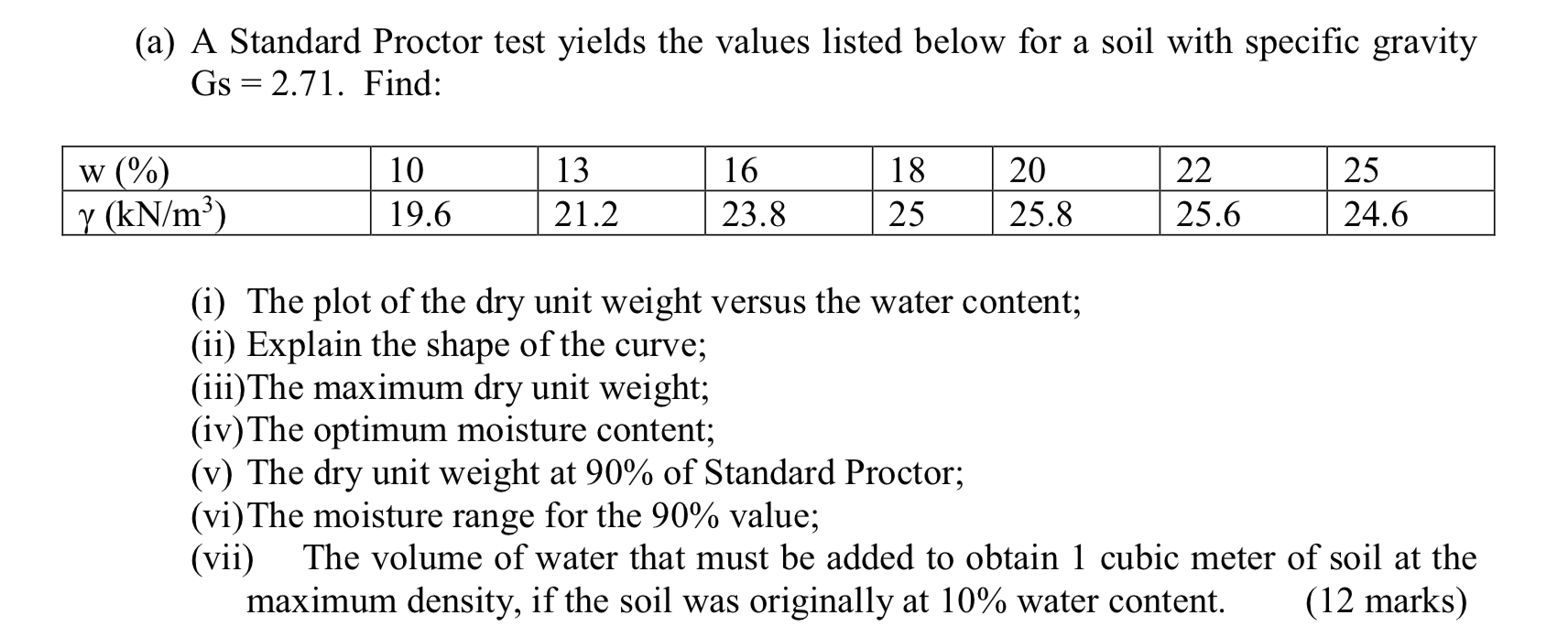# Question (a) A Standard Proctor test yields the values listed below for a soil with specific gravity Gs = 2.71. Find: 13 16 w (%) y (kN/m3) 10 19.6 18 25 20 25.8 22 25.6 25 24.6 21.2 23.8 (i) The plot of the dry unit weight versus the water content; (ii) Explain the shape of the curve; (iii) The maximum dry unit weight; (iv) The optimum moisture content; (v) The dry (a) A Standard Proctor test yields the values listed below for a soil with specific gravity Gs = 2.71. Find: 13 16 w (%) y (kN/m3) 10 19.6 18 25 20 25.8 22 25.6 25 24.6 21.2 23.8 (i) The plot of the dry unit weight versus the water content; (ii) Explain the shape of the curve; (iii) The maximum dry unit weight; (iv) The optimum moisture content; (v) The dry unit weight at 90% of Standard Proctor; (vi) The moisture range for the 90% value; (vii) The volume of water that must be added to obtain 1 cubic meter of soil at the maximum density, if the soil was originally at 10% water content. (12 marks)DTGIZO The Asker · Other NSMTranscribed Image Text: (a) A Standard Proctor test yields the values listed below for a soil with specific gravity Gs = 2.71. Find: 13 16 w (%) y (kN/m3) 10 19.6 18 25 20 25.8 22 25.6 25 24.6 21.2 23.8 (i) The plot of the dry unit weight versus the water content; (ii) Explain the shape of the curve; (iii) The maximum dry unit weight; (iv) The optimum moisture content; (v) The dry unit weight at 90% of Standard Proctor; (vi) The moisture range for the 90% value; (vii) The volume of water that must be added to obtain 1 cubic meter of soil at the maximum density, if the soil was originally at 10% water content. (12 marks)
More
Transcribed Image Text: (a) A Standard Proctor test yields the values listed below for a soil with specific gravity Gs = 2.71. Find: 13 16 w (%) y (kN/m3) 10 19.6 18 25 20 25.8 22 25.6 25 24.6 21.2 23.8 (i) The plot of the dry unit weight versus the water content; (ii) Explain the shape of the curve; (iii) The maximum dry unit weight; (iv) The optimum moisture content; (v) The dry unit weight at 90% of Standard Proctor; (vi) The moisture range for the 90% value; (vii) The volume of water that must be added to obtain 1 cubic meter of soil at the maximum density, if the soil was originally at 10% water content. (12 marks)